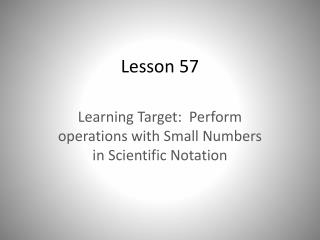DownloadDownload PresentationLesson 57

# Lesson 57

Download Presentation## Lesson 57

- - - - - - - - - - - - - - - - - - - - - - - - - - - E N D - - - - - - - - - - - - - - - - - - - - - - - - - - -
##### Presentation Transcript

1. Lesson 57 Learning Target: Perform operations with Small Numbers in Scientific Notation

2. Warm-up • 25(36) • Find the mean of 30 and 40 • 66 2/3 of \$300 • What is the probability of rolling a number greater than 1 with one roll of a number cube? • X/24 = 3/8 • Two angles of a parallelogram measure 80o and 80o. What are the measures of the other two angles?

3. Scientific Notation • 24,000,000 = • 0.000064 = • 13,000 = • 0.00000003 =

4. Rules of Exponents • Multiply Exponents – • ( 35 ) (37) = • (6-3 ) (65 ) = • Dividing Exponents – • (78 ) / (72 ) = • (9-4 ) / (9-8 ) = • Raising an Exponent to a power – • (42)5 = • (8-3)2 =

5. Example 1 • If a sheet of notebook paper is 0.01 cm thick, how tall is a stack of 2500 sheets of notebook paper? Express each number in scientific notation and perform the calculation in scientific notation.

6. Example 2 • (2.4 X 10-6) (2 X 10-2) • (2.4 X 10-6) / (2 X 10-2) • (4 X 10-6) (5 X 10-4) • (4 X 10-6) / (5 X 10-4)

7. Guided Practice • (4 X 1010) (2 X 10-6) • (1.5 X 10-5) (3 X 10-2) • (7.5 X 105) / (3 X 10-2) • (8.1 X 10-4) / (3 X 10-7)

8. BELL RINGER • Find the Product. • (3 X 104) (6 X 10-3) • Find the quotient. • (6 X 10-3) / (3 X 104)